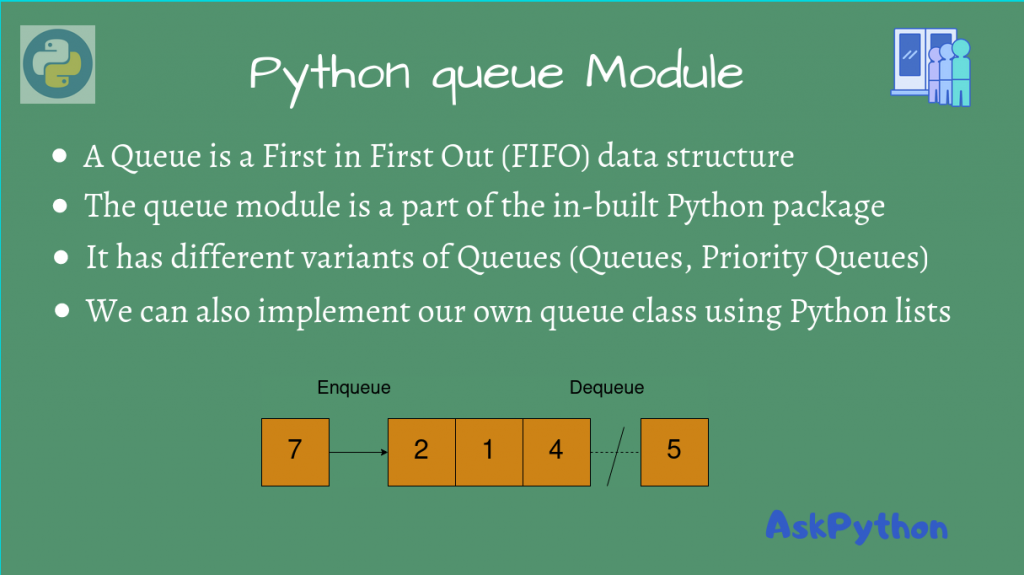# Python Queue ModuleIn this article, we shall look at the Python Queue module, which is an interface for the Queue data structure.

## Python Queue

A Queue is a data structure where the first element to be inserted is also the first element popped. It’s just like a real-life queue, where the first in line is also the first one out.

In Python, we can use the `queue` module to create a queue of objects.

This is a part of the standard Python library, so there’s no need to use `pip`.

Import the module using:

```import queue
```

To create a Queue object, we can instantiate it using:

```q = queue.Queue()
```

By default, this has a capacity of 0, but if you want to explicitly mention it, you can do so using:

```q = queue.Queue(max_capacity)
```

## Queue.get() and Queue.put() methods

We can insert and retrieve values into the Queue using the `queue.get()` and `queue.put()` methods.

Let’s create a queue and insert numbers from 1 to 5.

```import queue

# Instantiate the Queue object
q = queue.Queue()

# Insert elements 1 to 5 in the queue
for i in range(1, 6):
q.put(i)

print('Now, q.qsize() =', q.qsize())

# Now, the queue looks like this:
# (First) 1 <- 2 <- 3 <- 4 <- 5
for i in range(q.qsize()):
print(q.get())

```

Output

```Now, q.qsize() = 5
1
2
3
4
5
```

As you can see, the output shows that the first index is indeed 1, so that’s the top of the Queue. The rest of the elements follow it in a similar fashion.

## Empty a Python Queue

We can empty a queue object using `q.empty()`. This sets the size to 0, and empties the queue.

```import queue

# Instantiate the Queue object
q = queue.Queue()

# Insert elements 1 to 5 in the queue
for i in range(1, 6):
q.put(i)

print('Now, q.qsize() =', q.qsize())

# Empty queue
q.empty()

print('After emptying, size =', q.qsize())

for i in range(q.qsize()):
print(q.get())
```

Output

```Now, q.qsize() = 5
After emptying, size = 0

```

While most typical queue implementations have a `pop` (or `dequeue` operation), the `queue` module does not have a method for this.

So, if you want to pop elements from the queue, you must use a different queue class yourself. A simple solution would be to use Python’s list.

We’ll use `list.append(value)` to insert elements into the queue, since insertion happens at the end, and remove elements using `list.pop(0)`, since the first element is removed.

```class MyQueue():
# Using Python Lists as a Queue
def __init__(self):
self.queue = []

def enqueue(self, value):
# Inserting to the end of the queue
self.queue.append(value)

def dequeue(self):
# Remove the furthest element from the top,
# since the Queue is a FIFO structure
return self.queue.pop(0)

my_q = MyQueue()

my_q.enqueue(2)
my_q.enqueue(5)
my_q.enqueue(7)

for i in my_q.queue:
print(i)

print('Popped,', my_q.dequeue())

for i in my_q.queue:
print(i)

```

Output

```2
5
7
Popped, 2
5
7
```

We’ve written our own queue class with a `dequeue` operation! Now, we’ll show you how you could use other modules for using other types of Queues.

## Priority Queues in Python

Priority Queue is a type of queue that adds to the queue on the basis of an item’s priority, which is typically an integer value.

Items with a lower priority number are given a higher preference and are at the front of the queue, while others are behind.

The `queue` module also supports the Priority Queue structure, so let’s see how we can use it.

```import queue

priority_q = queue.PriorityQueue()

priority_q.put((1, 'Hello'))
priority_q.put((2, 'from'))

for i in range(priority_q.qsize()):
print(priority_q.get())
```

Output

```(1, 'Hello')
(2, 'from')
```

As you can see, elements are inserted on the basis of their priority.

## Python heapq Queue

We can also use the `heapq` module to implement our priority queues.

```>>> import heapq
>>> q = []
>>> heapq.heappush(q, (1, 'hi'))
>>> q
[(1, 'hi')]
>>> q
>>> heapq.heappush(q, (2, 'from'))
>>> q
[(1, 'hi'), (3, 'AskPython'), (2, 'from')]
>>> heapq.heappop(q)
(1, 'hi')
>>> heapq.heappop(q)
(2, 'from')
>>> heapq.heappop(q)
>>> heapq.heappop(q)
Traceback (most recent call last):
File "<stdin>", line 1, in <module>
IndexError: index out of range
```

So we are creating a priority queue and popping from it until it is empty. The same can also be achieved using the below program

```import heapq

q = []

heapq.heappush(q, (2, 'from'))
heapq.heappush(q, (1, 'Hello'))

while q:
# Keep popping until the queue is empty
item = heapq.heappop(q)
print(item)

```

Output

```(1, 'Hello')
(2, 'from')